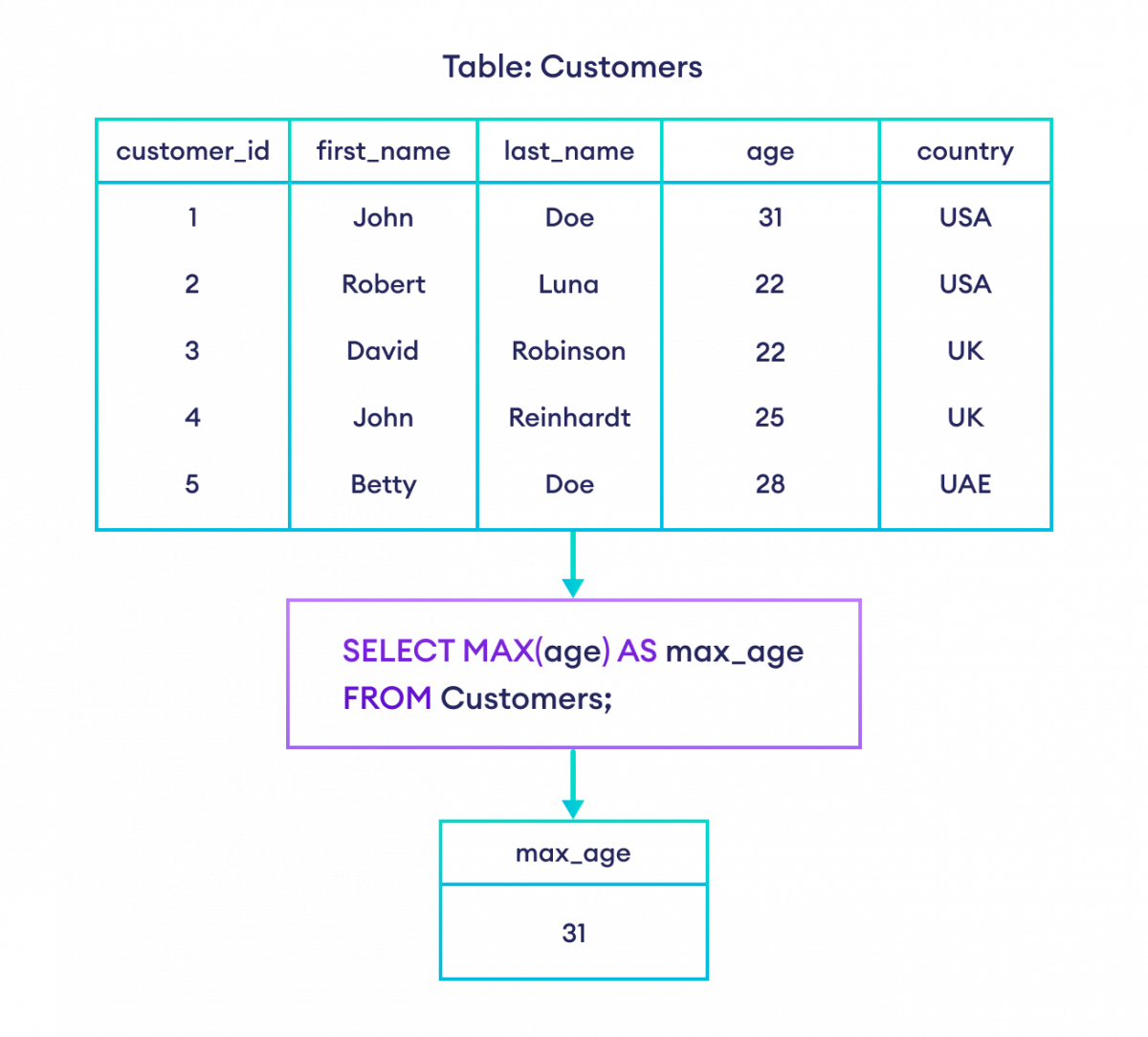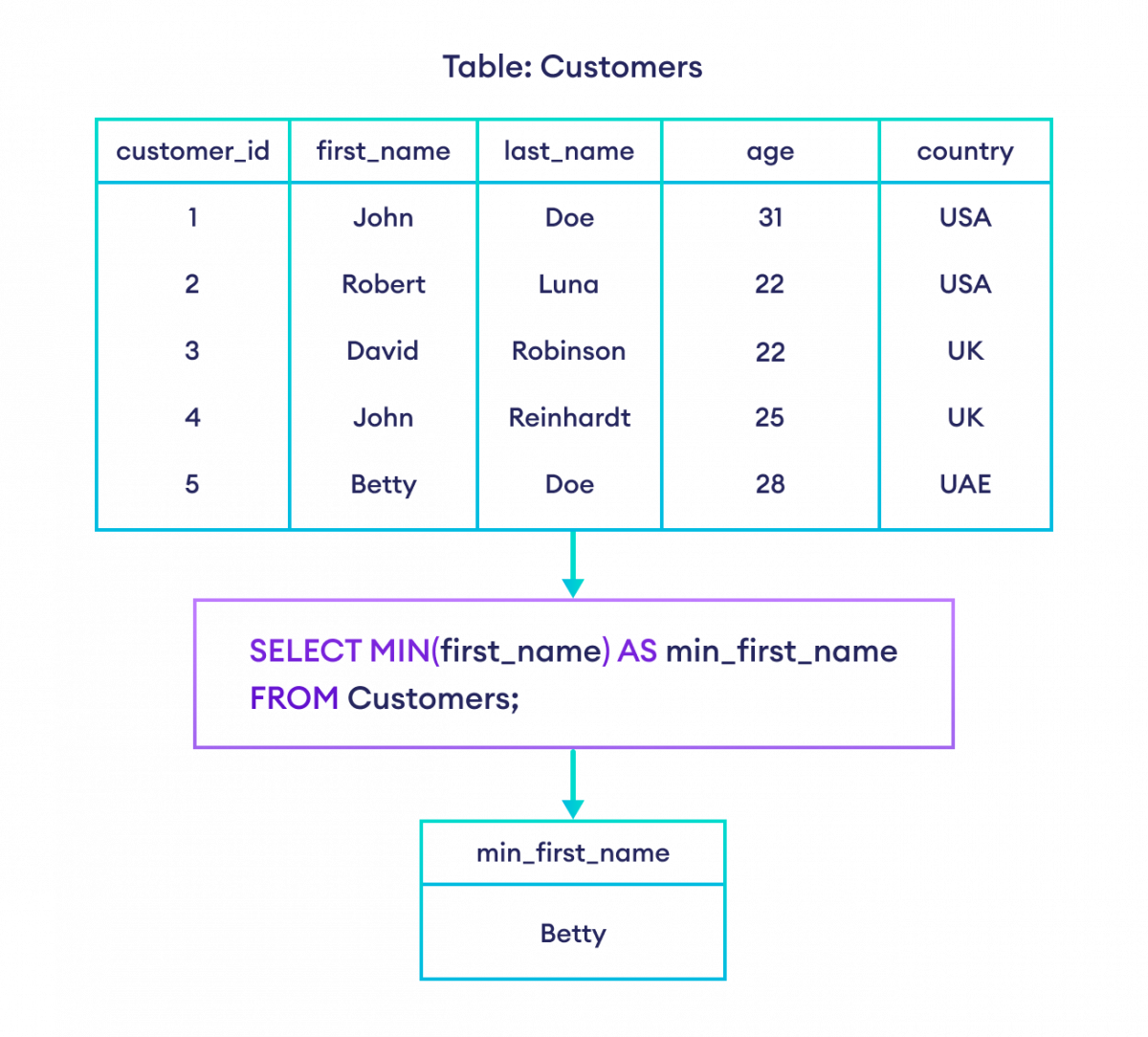# SQL MAX() and MIN()

In this tutorial, we'll learn about the MIN() and MAX() functions and how to use them with examples.

• The `MAX()` function returns the maximum value of a column.
• The `MIN()` function returns the minimum value of a column.

## 1. SQL MAX() Function

Let's take an example.

``````SELECT MAX(age)
FROM Customers;``````

Here, the SQL command returns the largest value from the age column.

## 2. SQL MIN() Function

Let's take an example.

``````SELECT MIN(age)
FROM Customers;``````

Here, the SQL command returns the smallest value from the age column.

## Aliases with MAX() and MIN()

In the above examples, the field name in the result set is `MIN(age)` and `MAX(age)`.

It is also possible to give custom names to these fields using the `AS` keyword. For example,

``````SELECT MAX(age) AS max_age
FROM Customers;``````

Here, the field name `MAX(age)` is replaced with max_age in the result set.Example: MAX() in SQL with Alias

## MAX() and MIN() with Strings

The `MAX()` and `MIN()` functions also work with other data types such as text, not just numbers. For example,

``````SELECT MIN(first_name) AS min_first_name
FROM Customers;``````

Here, the SQL command selects the minimum value of `first_name` based on the dictionary order.Example: MIN() in SQL with String

## Select Row Containing Max/Min Value

If we need to select the entire row(s) containing max/min value, we can use the nested `SELECT` statement in this way:

``````-- Get entire rows containing the minimum age
SELECT *
FROM Customers
WHERE age = (
SELECT MIN(age)
FROM Customers
);``````

Here, the SQL command selects all the rows having the lowest age value.

## More SQL MAX() and MIN() Examples

MAX() and MIN() of Two or More Values

We can also find the highest or lowest value from two or more values using the MAX() and MIN() function. For example,

``````-- returns 50 as highest
SELECT MAX(20, 30, 50) as highest;

-- returns 20 as lowest
SELECT MIN(20, 30, 50) as lowest;``````
MAX() and MIN() with HAVING

Let's take an another example,

``````SELECT *
FROM Customers
GROUP BY country
HAVING MAX(age);``````

Here, the SQL command returns max age in each country from the Customers table.

To learn more, visit SQL HAVING Clause.